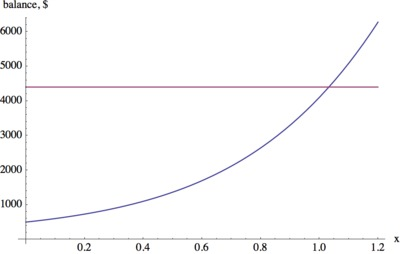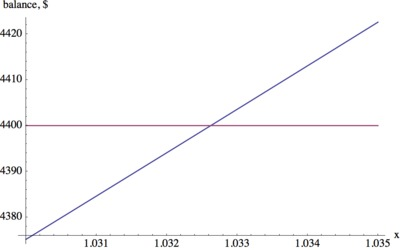# Introduction to Polynomials - College Fund

Alignments to Content Standards: A-REI.D.11 A-CED.A.1

When Marcus started high school, his grandmother opened a college savings account. On the first day of each school year she deposited money into the account: \$1000 in his freshmen year, \$600 in his sophomore year, \$1100 in his junior year and \$900 in his senior year. The account earns interest of $r\%$ at the end of each year. When Marcus starts college after four years, he gets the balance of the savings account plus an extra \$500. 1. If$r$is the annual interest rate of the bank account, the at the end of the year the balance in the account is multiplied by a growth factor of$x=1+r$. Find an expression for the total amount of money Marcus receives from his grandmother as a function of this annual growth factor$x.$2. Suppose that altogether he receives \$4400 from his grandmother. Use appropriate technology to find the interest rate that the bank account earned.
3. How much total interest did the bank account earn over the four years?
4. Suppose the bank account had been opened when Marcus started Kindergarten. Describe how the expression for the amount of money at the start of college would change. Give an example of what it might look like.

## IM Commentary

This task could serve as an introduction to polynomials or as an application after students are familiar with this type of function.

As an introduction to polynomials the first part of the task could be done as a classroom discussion. If students are already familiar with exponential functions, the set up of the task, i.e. the balance in a bank account, might seem familiar. This task illustrates that if we vary the interest rate rather than the time we get a different type of function to model the balance in a bank account.

Students may need some guidance with respect to the "change of variables" $x=1+r$ and its purpose. After the class has come up with the polynomial function, students can use the function to answer the main questions about the interest rate and the interest earned individually or in groups. The last part of the task is more open ended and groups could share their solutions and explain the reasoning that led them to specific examples. This discussion provides a nice opportunity to reinforce the meaning of the different terms in the expression.

If the task is used later in a unit on polynomials, students can work on it more independently and share their solutions at the end.

This task lends itself to engage in several mathematical practices: SMP 5 "Use appropriate Tools Strategically" and SMP 8 "Look For and Express Regularity in Repeated Reasoning".

## Solution

1. After the first deposit, the balance is \$1000. After one year, we earn interest on this amount, so it will grow to$\$1000(1+r)$. If we let $x=1+r$ we have $\$1000x$. Since Marcus' grandmother also deposits an additional \$600, so we have a total of $1000x +600$ dollars in the account after one year.

Afte another year, the amount already in the account will earn interest and it will grow to $(1000x+600)x=1000x^2+600x$. We also have an additional deposit of \$1100, so the balance after 2 years is$1000x^2+600x+1100$. After 3 years the money in the account will grow to$(1000x^2+600x+1100)x = 1000x^3+600x^2+1100x$dollars. With the additional deposit of \$900 the total balance is $1000x^3+600x^2+1100x+900$ dollars.

After 4 years we gain interest again on the previous balance and add the final present of \$500: \begin{eqnarray*} p(x)&=&(1000x^3+600x^2+1100x+900)x +500\\ &=&1000x^4 + 600 x^3 + 1100 x^2 + 900 x + 500 \text{ dollars}. \end{eqnarray*} 2. We can use a graphing calculator or a computer algebra system to graph$y=p(x)$. Since$p(x)$represents the balance after 4 years if the growth rate is$x$, we can graph$p(x)$and find which value of$x$corresponds to a balance of 4400. As in the graph below, this can be visualized as the point where$p(x)$crosses the horizontal line.We see that for$x$between 1 and 1.05 the balance reaches \$4400. Zooming in on that part of the graph we find that for $x\approx 1.0326$ we have $p(x) = 4400$. So this means that when the growth factor is 1.0326 the balance will grow to \$4400 in four years. Since$x=1+r$, where$r$is the interest rate, the bank account earns$r=0.0326= 3.26\%$interest annually.We can also use a graphing calculator or CAS to solve$p(x) = 4400$for$x$to obtain$x=-1.47734$,$x=-0.0776439 - 1.59701 i$,$x=-0.0776439 + 1.59701 i$and$x=1.03263.$Below is a screenshot if we use WolframAlpha:Since in the context of the problem we know that the growth factor has to be a positive real number, we find that the solution is$x=1.03263.$Therefore, the interest rate of the savings account is$r=3.263\%.$3. Marcus' grandmother made total deposits and gifts of$\$(1000+600+1100+900+500)= \$4100.$Since Marcus receives \$4400, the additional \$300 must be the earned interest. 4. If Marcus' grandmother had started the savings account when Marcus started kindergarten, the process until Marcus started college would have taken 13 years. Without knowing the yearly deposits we can still say that the expression for the amount of money at the end as a function of the interest growth factor would have been $$a_{13}x^{13}+a_{12}x^{12}+a_{11}x^{11}+\cdots + a_1x+a_0,$$ where$a_{13}$is the first deposit,$a_{12}$is the second deposit etc., and$a_0$is the final gift. If each deposit had been \$200 the expression would be: $$200x^{13}+200x^{12}+200x^{11}+\cdots + 200x+200,$$